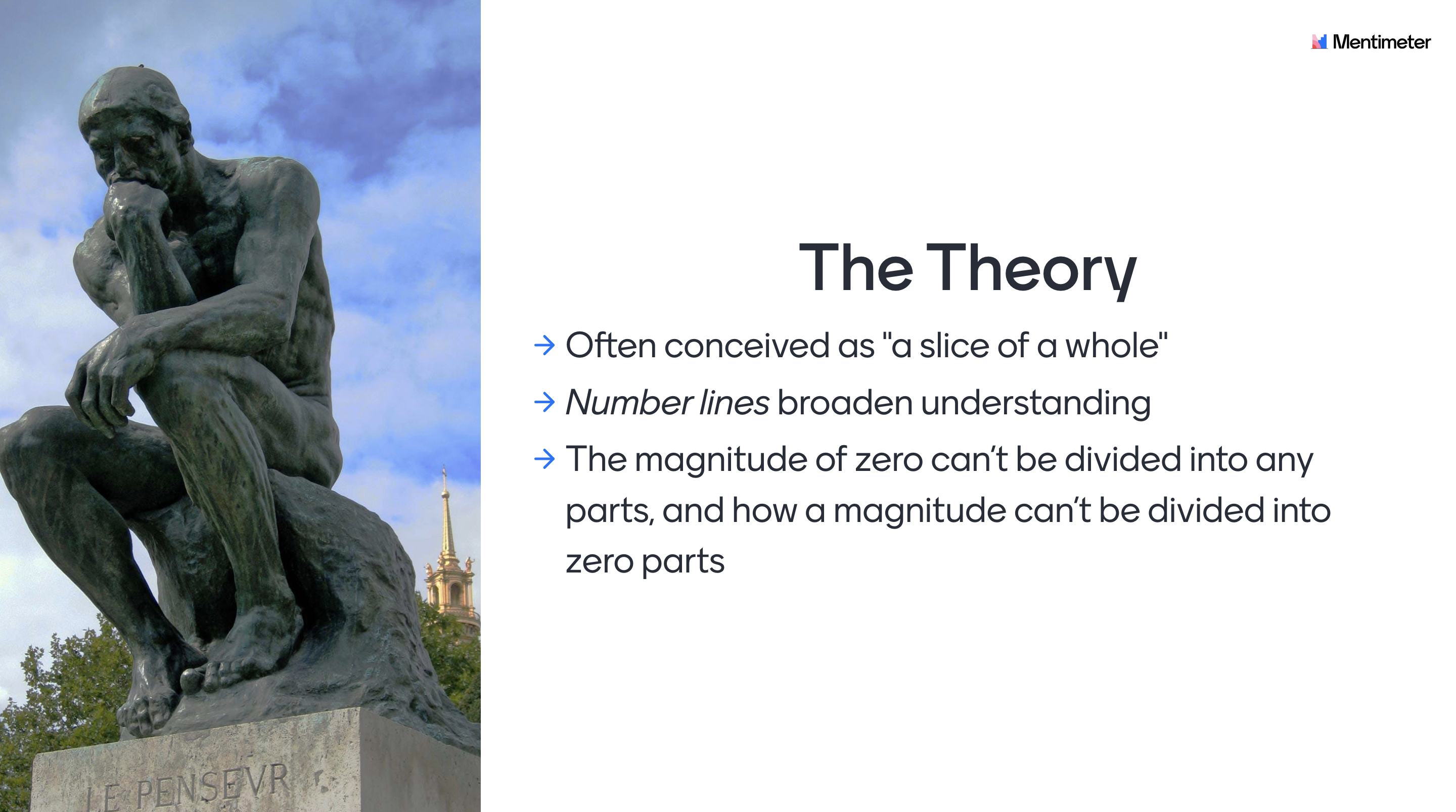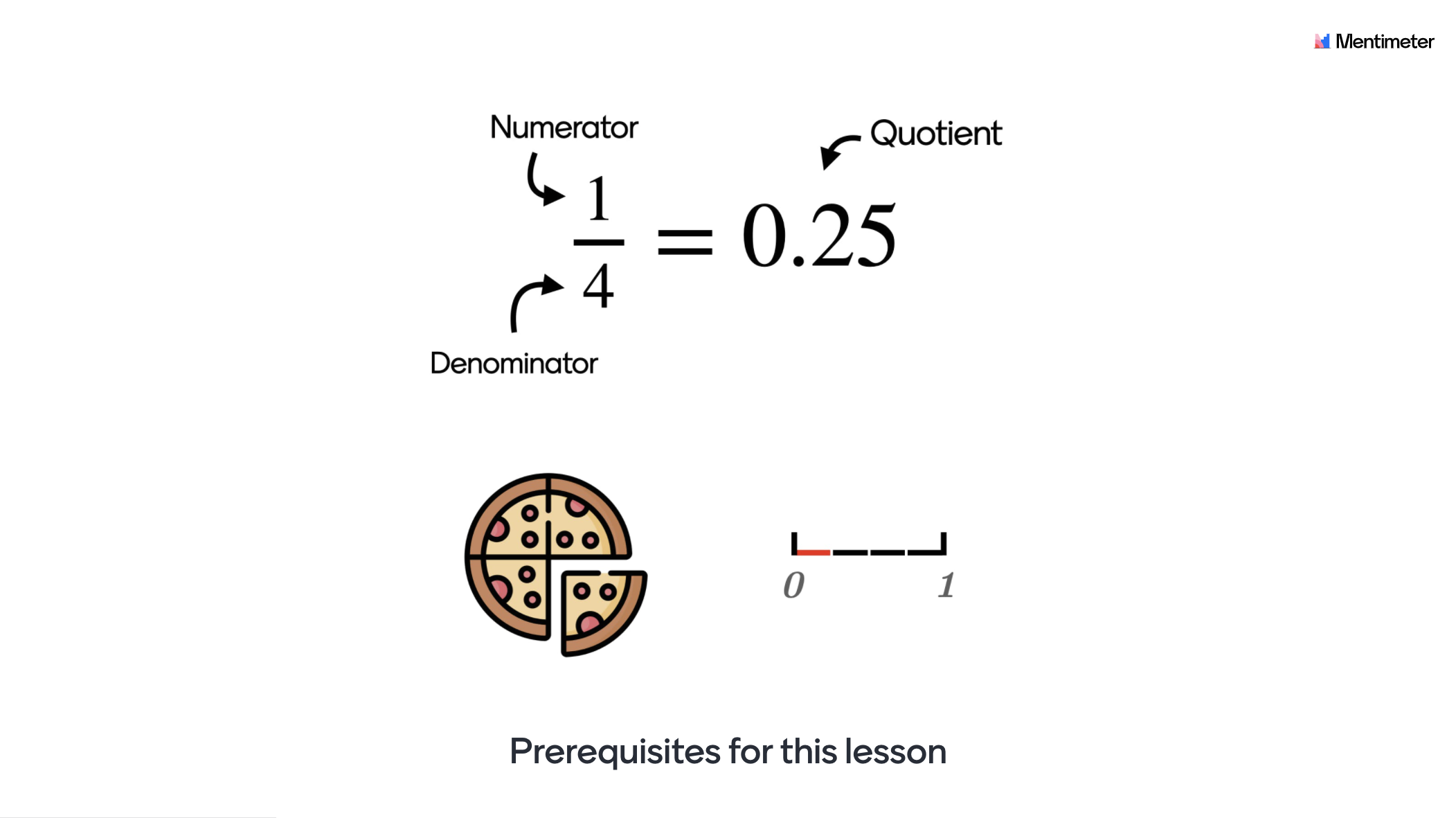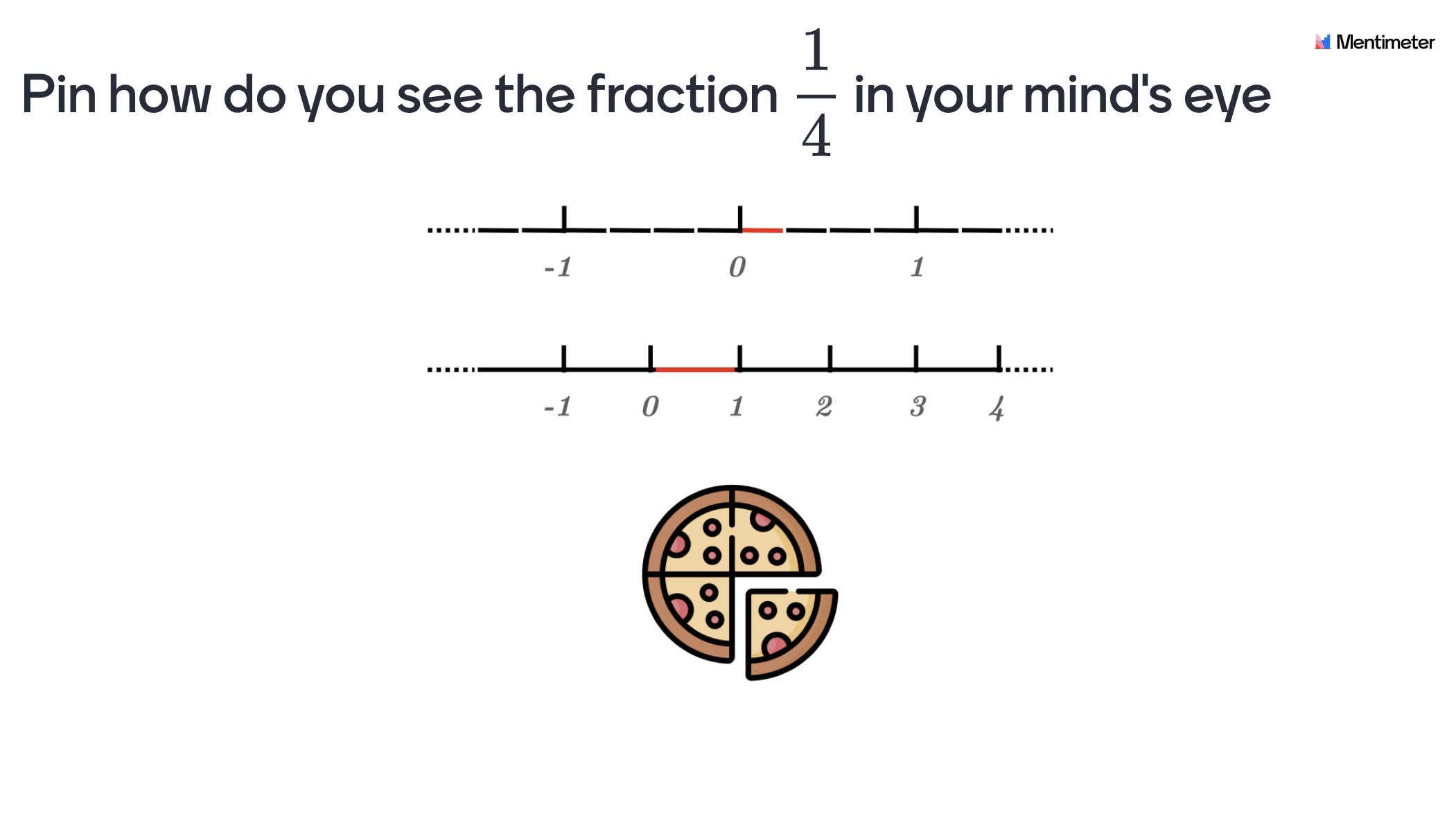# The Concept of Fractions

This Mentimeter template elaborates with the concept of fractions and the intended target group is students in 6th grade. The template is designed foremost as an introduction but can also be used in the middle or as a form of reinforcement at the end of a subject block surrounding fractions.## How to use the template

The students need a pencil and paper for some of the questions in the presentation.

Here follows some ideas on how the slides are intended to be used but feel free to use the template however you like. Included in each cell is the purpose and other information that could be of use for you as a teacher. A general idea regarding all questions is that the students should work after the model Think-Pair-Share (TPS). When implementing TPS the students should first answer the question individually, then discuss in pairs (or groups of three) followed by a classroom discussion. As a concluding remark. The content in the slides are designed primarily for developing the students understanding of mathematical concepts rather than the procedural 'know how'. Therefore discussions and questions from the students is a central part of using the presentation where your expertise as a teacher is central to clarify misconceptions and lead the classroom discussions.Fractions are a part of every person's life and even children need to learn how to divide stuff equally among their friends. Research has shown that fractions are often conceived as "a slice of a whole" but to obtain a deeper understanding for the conceptual for fraction, one way is to work with magnitude understanding presented within number lines. Number lines could according to Gersten, et al. (2017) broaden the understanding of fractions and for example shed light on why the magnitude of zero can’t be divided into any parts, and how a magnitude can’t be divided into zero parts. In other words why we are allowed to have zero in the numerator but not in the denominator.Slide 1 - Introduction: Introduce the theme of the lecture and present the learning goals. For example you could mention that fractions is an essential part of mathematics that have many practical implications.

Slide 2 - General information: Inform the students regarding the TPS methodology and how they can access the interactive presentation.

Slide 3 - Criteria for success: Introduce a "criteria for success" for the lesson with a problem that will be revisited and solved at the end of the lesson. The idea here is to make the learning visible, so the students can see for themselves that they’ve actually learned something.

Slide 4 - According to the students, a fraction is...: Let the students individually write down how they see the concept of fractions. This can help clarify potential misconceptions.

Slide 5 - Prerequisites for this lesson: Introduce what the numerator, denominator and quotient is.Slide 6 - A fraction as a "slice of a whole" or as "part of a number line": Research has shown that it is beneficial to be able to see a fraction represented by a number line. This slide aims to introduce that a fraction can be represented in different ways.

Slide 7 - What happens to the quotient when the numerator increases?: Here the aim is that the students should start to understand what an increase or decrease of the numerator means for the quotient.

Slide 8 - What happens to the quotient when the denominator increases?: Here the aim is that the students should start to understand what an increase or decrease of the denominator means for the quotient.

Slide 9 - Rank fractions: Let the students rank the fractions by order of magnitude. This can help them consolidate their newly found understanding of fractions, and lets you as a teacher see potential misconceptions.

Slide 10 - If x and y are positive and x>y, then x/y is always…: A continuation from the previous two slides. This is a good chance to evaluate if the students have grasped what it means when the numerator is larger than the denominator.Slide 11 - If you have any positive number x, and divide it by y, 0<y<1, what is true about the quotient?: The idea here is to realize that whatever number you choose on x, you can only get a quotient that is bigger than x itself. Because you always divide x into smaller parts than what x consists of as a whole.

Slide 12 - What is the difference between dividing by a whole number  and a fraction?: Give a visual representation using the number line of what it means to divide by a fraction.

Slide 13 - Practice dividing by a fraction using the number line: Let the students practice on using the number line to solve fraction division.

Slide 14 - Criteria for success revisited: Return to the problem that was introduced in the beginning of the lecture to make the students realize they’ve actually learnt something.

Slide 15 - Q&A: Let the students individually write down what they still feel is unclear about fractions.

Slide 16-22 - Quiz competition!: Ask the students to enter their real names. Let the students compete in a fun way while you as a teacher can get a sense of the individual level of knowledge. This information could be used to give support for students in need of extra guidance.